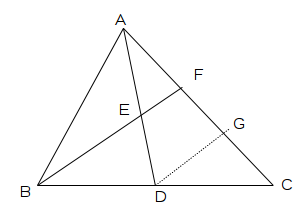### In a triangle ABC, AD is the median. F is the point on AC such that line BF bisect AD at E. Prove that AC = 3AF.

Step by Step Explanation:
1. Following picture shows triangle ΔABC with median AD at E, and another line BF, which bisects AD. We have also drawn another line DG which is parallel to BF.2. From proportionality theorem we know that if a line is drawn parallel to one side of a triangle, it divides other two sides in the same ration.
3. For triangle ΔBFC, DG is parallel to BF and using proportionality theorem we can find that,

 FG GC
=
 BD DC

4. Since we know that BD = DC, we can find that,
GC = FG ---------(1)
5. Similarly we can use proportionality theorem for triangle ΔADG,

 AF FG
=
 AE ED

6. But since AE = ED,
FG = AF ---------(2)
7. Therefore,
AC = AF + FG + GC
Using relation (1) and (2),
AC = AF + AF + AF,
AC = 3AF